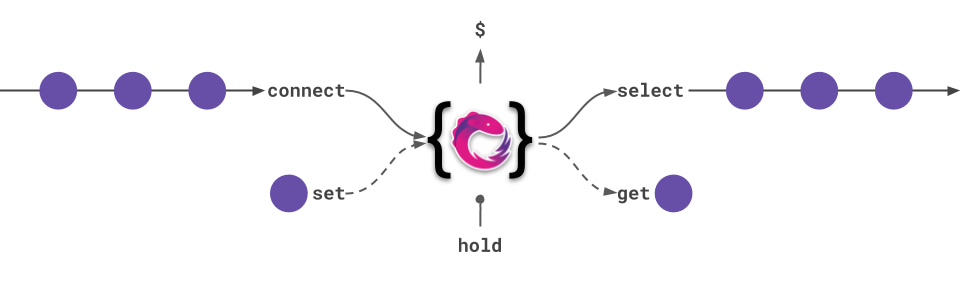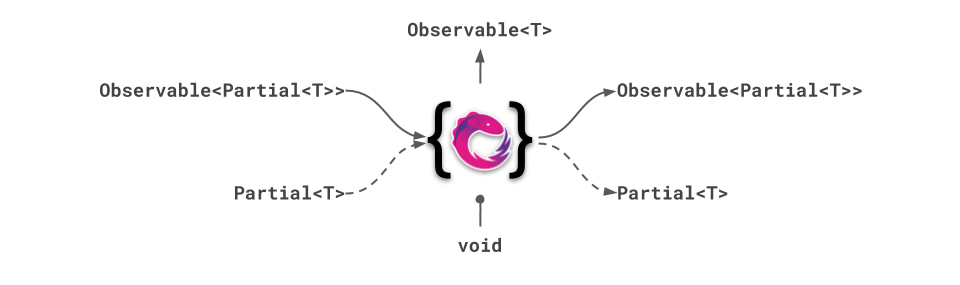# RxState

## Overview​

RxState is a light-weight reactive state management service for managing local state in angular.

Example

``Component({  selector: 'app-stateful',  template: `<div>{{ state\$ | async | json }}</div>`,  providers: [RxState],});export class StatefulComponent {  readonly state\$ = this.state.select();  constructor(private state: RxState<{ foo: string }>) {}}``

## Signature​

``class RxState<T extends object> implements OnDestroy, Subscribable<T> {  readonly \$: Observable<T> = this.accumulator.signal\$;  connect(inputOrSlice\$: Observable<Partial<T> | V>, projectFn?: ProjectStateReducer<T, V>) => void;  connect(key: K, slice\$: Observable<T[K]>) => void;  connect(key: K, input\$: Observable<V>, projectSliceFn: ProjectValueReducer<T, K, V>) => void;  set(stateOrProjectState: Partial<T> | ProjectStateFn<T>) => void;  set(key: K, projectSlice: ProjectValueFn<T, K>) => void;  select(op: OperatorFunction<T, A>) => Observable<A>;  select(k1: K1) => Observable<T[K1]>;  select(k: K, fn: (val: T[K]) => V): Observable<V>;  select(keys: K[], fn: (slice: PickSlice<T, K>) => V, keyCompareMap?: KeyCompareMap<Pick<T, K>>): Observable<V>;  select() => Observable<T>;  get() => T;  get(k1: K1) => Partial<T>;  hold(obsOrObsWithSideEffect: Observable<S>, sideEffectFn?: (arg: S) => void) => void;  setAccumulator(accumulatorFn: AccumulationFn) => void;}``## \$ (state observable)​

##### typeof: Observable<T>​

The unmodified state exposed as `Observable<T>`. It is not shared, distinct or gets replayed. Use the `\$` property if you want to read the state without having applied stateful to it.

## connect​

### Signature​

``connect(inputOrSlice\$: Observable<Partial<T> | V>, projectFn?: ProjectStateReducer<T, V>): void``

Connect an `Observable<Partial<T>>` to the state `T`. Any change emitted by the source will get merged into the state. Subscription handling is done automatically.

Example

``const sliceToAdd\$ = interval(250).pipe(mapTo({  bar: 5,  foo: 'foo'});state.connect(sliceToAdd\$);// every 250ms the properties bar and foo get updated due to the emission of sliceToAdd\$// Additionally you can provide a `projectionFunction` to access the current state object and do custom mappings.const sliceToAdd\$ = interval(250).pipe(mapTo({  bar: 5,  foo: 'foo'});state.connect(sliceToAdd\$, (state, slice) => state.bar += slice.bar);// every 250ms the properties bar and foo get updated due to the emission of sliceToAdd\$. Bar will increase by// 5 due to the projectionFunction``

### Signature​

``connect(key: K, slice\$: Observable<T[K]>): void``

Connect an `Observable<T[K]>` source to a specific property `K` in the state `T`. Any emitted change will update this specific property in the state. Subscription handling is done automatically.

Example

``const myTimer\$ = interval(250);state.connect('timer', myTimer\$);// every 250ms the property timer will get updated``

### Signature​

``connect(key: K, slice\$: Observable<V>, projectSliceFn: ProjectValueReducer<T, K, V>): void``

Connect an `Observable<V>` source to a specific property in the state. Additionally you can provide a `projectionFunction` to access the current state object on every emission of your connected `Observable`. Any change emitted by the source will get merged into the state. Subscription handling is done automatically.

Example

``const myTimer\$ = interval(250);state.connect(  'timer',  myTimer\$,  (state, timerChange) => (state.timer += timerChange));// every 250ms the property timer will get updated``

## set​

### Signature​

``set(stateOrProjectState: Partial<T> | ProjectStateFn<T>): void``

Manipulate one or many properties of the state by providing a `Partial<T>` state or a `ProjectionFunction<T>`.

Example

``// Update one or many properties of the state by providing a `Partial<T>`const partialState = {  foo: 'bar',  bar: 5,};state.set(partialState);// Update one or many properties of the state by providing a `ProjectionFunction<T>`const reduceFn = (oldState) => ({  bar: oldState.bar + 5,});state.set(reduceFn);``

### Signature​

``set(key: K, projectSlice: ProjectValueFn<T, K>): void``

Manipulate a single property of the state by the property name and a `ProjectionFunction<T>`.

Example

``const reduceFn = (oldState) => oldState.bar + 5;state.set('bar', reduceFn);``

## select​

### Signature​

``select(): Observable<T>``

Returns the state as cached and distinct `Observable<T>`. This way you don't have to think about late subscribers, multiple subscribers or multiple emissions of the same value

Example

``const state\$ = state.select();state\$.subscribe((state) => doStuff(state));``

### Signature​

``select(op: OperatorFunction<T, A>): Observable<A>``

Returns the state as cached and distinct `Observable<A>`. Accepts arbitrary rxjs operators to enrich the selection with reactive composition.

Example

``const profilePicture\$ = state.select(  pluck('profilePicture'),  switchMap((profilePicture) => mapImageAsync(profilePicture)));``

### Signature​

``select(k1: K1): Observable<T[K1]>``

Access a single property of the state by providing keys. Returns a single property of the state as cached and distinct `Observable<T[K1]>`.

Example

``// Access a single propertyconst bar\$ = state.select('bar');// Access a nested propertyconst foo\$ = state.select('bar', 'foo');``

### Signature​

``select(k: K, fn: (val: T[K]) => V): Observable<V>;``

Transform a single property of the state by providing a key and map function. Returns result of applying function to state property as cached and distinct `Observable<V>`.

Example

``// Project state based on single propertyconst foo\$ = state.select('bar', (bar) => `bar equals \${bar}`);``

### Signature​

``select(keys: K[], fn: (slice: PickSlice<T, K>) => V, keyCompareMap?: KeyCompareMap<Pick<T, K>>): Observable<V>;``

Transform a slice of the state by providing keys and map function. Returns result of applying function to state slice as cached and distinct `Observable<V>`.

Example

``// Project state sliceconst text\$ = state.select(  ['query', 'results'],  ({ query, results }) => `\${results.length} results found for "\${query}"`);``

## get​

### Signature​

``get(): T``

Read from the state in imperative manner. Returns the state object in its current state.

Example

``const { disabled } = state.get();if (!disabled) {  doStuff();}``

### Signature​

``get(k1: K1): Partial<T>``

Read from the state in an imperative manner by providing keys as parameters to reach deeply nested values. Returns the part of state object.

Example

``interface State {  bar: { foo: `test` };  baz: true;}// Access a single propertyconst bar = state.get('bar');// Access a nested propertyconst foo = state.get('bar', 'foo');``

## hold​

### Signature​

``  hold(obsOrObsWithSideEffect: Observable<S>, sideEffectFn?: (arg: S) => void): void``

Manages side-effects of your state. Provide an `Observable<any>` side-effect and an optional `sideEffectFunction`. Subscription handling is done automatically.

Example

``// Directly pass an observable side-effectconst localStorageEffect\$ = changes\$.pipe(  tap((changes) => storeChanges(changes)));state.hold(localStorageEffect\$);// Pass an additional `sideEffectFunction`const localStorageEffectFn = (changes) => storeChanges(changes);state.hold(changes\$, localStorageEffectFn);``

## setAccumulator​

### Signature​

``setAccumulator(accumulatorFn: AccumulationFn) => void``

Allows to customize state accumulation function. This can be helpful to implement deep updates and tackle other immutability problems in a custom way.

Example

``const myAccumulator = (state: MyState, slice: Partial<MyState>) => ({  ...state,  ...slice,});this.state.setAccumulator(myAccumulator);``